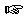### Section 10

Problem 1:

A sound processor filters out frequencies below a low frequency threshold and above a high frequency threshold. Such a processor might be used as part of an MP3 encoder to reduce the amount of data to be processed. Given that a frequency is a number, develop the function, filter-freq, which consumes two threshold frequencies (low and high) and a list of frequencies and returns a list of those frequencies between and including the thresholds.Solution

Problem 2:

Develop a data definition for a list of symbols and numbers.

Develop the function symbol-count, which consumes a list of symbols and numbers and returns the number of symbols in the list.Solution

Problem 3:

An auction bid contains three pieces of information: bidder number, item number, and amount of bid.

Develop a structure and data definition for an auction bid. Develop a data definition for a list of auction bids.

Develop the function highest-bid, which consumes an item number and a non-empty list of auction bids and returns the winning bid for that item. Assume that at least one bid for the item in question is contained in the list.Solution

Problem 4:
A Substance is a Symbol.

A Tank is a structure

`(make-tank Substance Number)`

`(define-struct tank (subst liters))`

A storage company would like to consolidate the contents of each tank holding the same substance into a single tank for each substance.

Develop the function remove, which consumes a substance, such as 'oil, and a list of tanks, and removes any tanks containing the substance from the list.

Develop the function combine, which consumes a substance S and a list of tanks. It produces the sum of all S found in the list.

Develop the function consolidate, which consumes a list of tanks, some of which may contain the same substance, and produces a list containing only one tank for each substance. This function should ensure that total amount of each substance in the new list is the same as the total amount of each substance in the original list.
Warning: This requires a (simple) generative recursion.Solution

Problem 5:
An SB is a list of two items: `(cons String (cons Boolean empty))`

An SB-list is either
- `empty`
- `(cons SB SB-list)`

Develop the function which-toppings, which consumes an SB-list and produces the list of strings that are associated with `true`.Solution

 Jamie Raymond Matthias Felleisen 01 december 2003